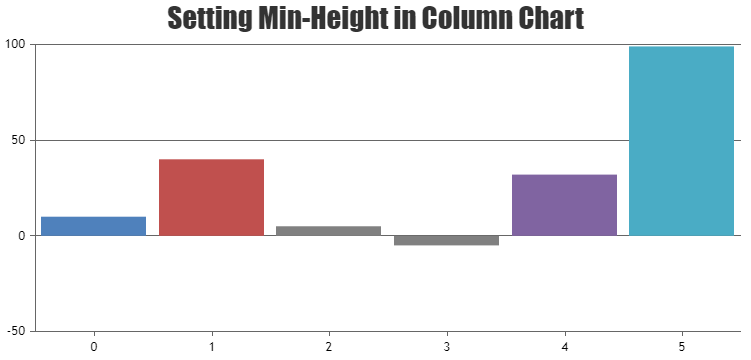Home Forums Chart Support When the magnitude between values are too high not all the columns appear Reply To: When the magnitude between values are too high not all the columns appear

#35945

Sorry, It is not possible to set minimum height of the column. However you can set minimum height to the columns with few lines of code as shown in the below code snippet

``````function setMinValue() {
var data = chart.options.data;
for(var i = 0; i < data.length; i++) {
for(var j =0; j < data[i].dataPoints.length; j++) {
var yValue = data[i].dataPoints[j].y;
if(yValue <= minValue && yValue >= -minValue) {
data[i].dataPoints[j].color = "gray";
data[i].dataPoints[j].y = yValue < 0 ? -minValue : minValue;
data[i].dataPoints[j].toolTipContent = ("{x}: " + yValue);
}
}
}
}``````

Also take a look at this JSFiddle for complete code.Ranjitha R
Team CanvasJS